M E N U
Close

 註冊／信箱驗證 | 登入

Close

Calculus 4 Using Polar Coordinates And Polar Equations 極坐標以及極坐標方程的運用

4.01 個評分7  /  7 Videos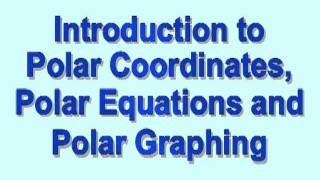1.  Polar Coordinates, Polar Equations, and Polar Graphs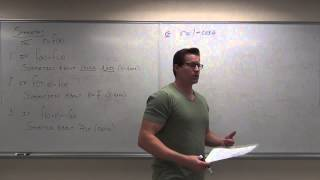2.  Calculus 2 Lecture 10.4: Using Polar Coordinates and Polar Equations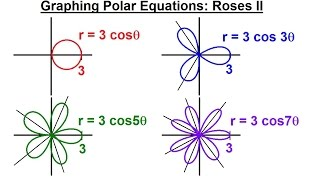3.  PreCalculus - Polar Coordinates (15 of 35) Graphing Polar Equations: r=3cos3(theta), Roses4.  Polar coordinates 1 | Parametric equations and polar coordinates | Precalculus | Khan Academy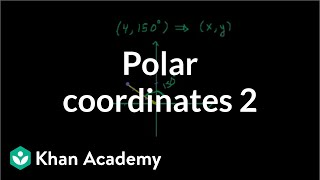5.  Polar coordinates 2 | Parametric equations and polar coordinates | Precalculus | Khan Academy6.  Polar coordinates 3 | Parametric equations and polar coordinates | Precalculus | Khan Academy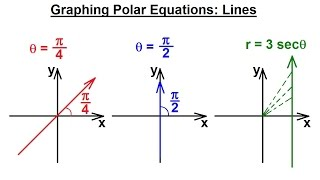7.  PreCalculus - Polar Coordinates (13 of 35) Graphing Polar Equations: theta=pi/4, theta=pi/4, Lines
• 頻道簡介
• 留言討論
• 新增影片
• 建議下架
• 最新消息
• 資訊交流

### All videos in this website are hosted and uploaded to video-sharing platform YouTube. SwiTube provides video playlists as a service to the public, it does not own, represent nor imply any association or affiliation with respective content.